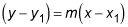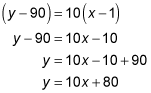##### Common Core Math For Parents For Dummies with Videos OnlineIn the Common Core program, eighth grade algebra is mostly linear. Linear relationships, which are equations with straight-line graphs, can include the slope-intercept form, standard form, and point-slope form.

A proportional relationship has a graph that makes a straight line and goes through the point (0,0). The graph of a linear relationship can go through the point (0,0) but doesn't have to. A linear relationship does have to have a constant rate of change between the two variables.

Linear relationships can appear in any of the following forms. You can transform any one of these forms into the others, and different forms have different purposes:

• Slope-intercept form: The slope-intercept form, represented as y = mx + b, is useful for describing situations involving change or movement.

For example, if your little brother runs 10 feet per second and you give him an 80-foot head start in a race, then you can describe his distance from the starting line (y) as y = 10x + 80. Your little brother's head start appears as the value of y when x is zero. His running speed appears as the coefficient of x. (A coefficient is a number that is multiplied by a variable; 10x means ten times x, so you can say that 10 is the coefficient of x.)

• Standard form: Standard form, represented as ax + by = c, is the more natural form for situations where the two variables are being added together.

For example, if you and a friend eat lunch at a restaurant together, and your total bill is \$33.18, you can write x + y = 33.18, where x represents their part of the bill and y represents your part of the bill. This equation represents all possible combinations of your lunch costs for this particular total. If you add a constraint, such as that their lunch cost twice as much as yours — written as x = 2y or x – 2y = 0 — then the two equations together form a system with (in this case) a single solution.

• Point-slope form: Point-slope form, represented asis a lot like slope-intercept form, except that it assumes you know a point other than the starting point.

Say that you knew that your brother was 90 feet from the starting line after 1 second, but you didn't know how big his head start was. Then you could write (y – 90) = 10 (x – 1). After a little algebraic manipulation:This equation comes out the same as the one in point-slope form: y = 10x + 80. The two forms are equivalent.

Linear equations come in several forms, and these forms have different uses. But the hallmark of a linear equation (or linear function) is that there is a constant rate that relates the two variables — that is, when the value of one variable changes by a certain amount, the other variable changes by a certain amount, and the ratio of these amounts is unchanging. In the younger-brother-running-race example, the rate is feet per second. In the first restaurant bill example, x + y = 33.18, the rate is disguised but still there. If your bills add to a total of \$33.18, then every dollar more that they spend on lunch is one dollar less that you spend. So there is a rate of –1 dollar on your bill per dollar on their bill.